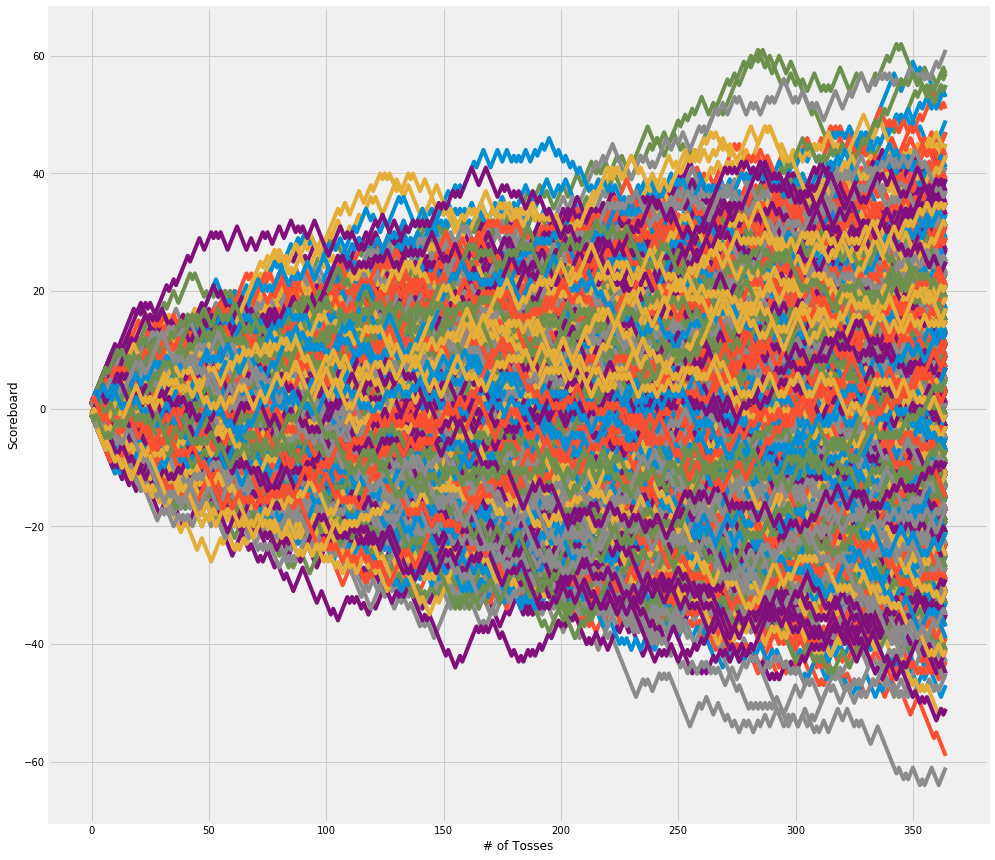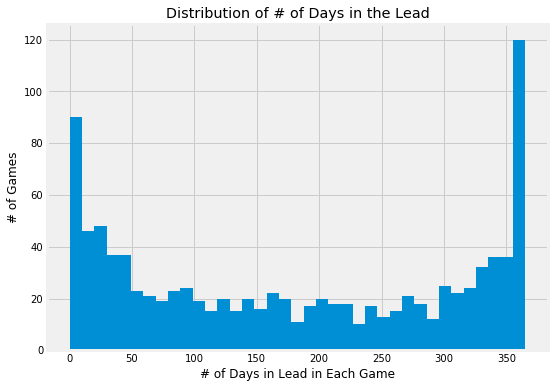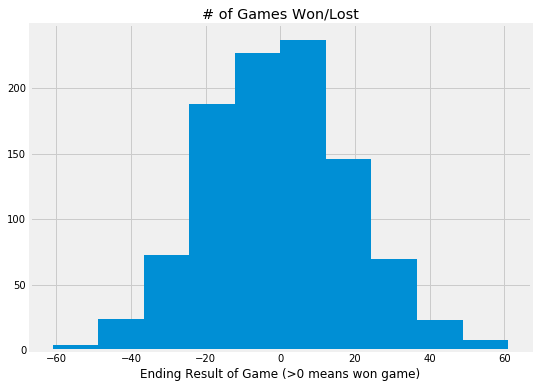# Random Walks and Gambler's Ruin - Fair Coin Tosses in Sequence

Posted 2018-12-24

## Random Walk and Gambler's Ruin¶

• Given a fair coin with repeated independent tosses in sequences, it leads to a surprising result of dominate winners.
• One would intuitively expect a normal distribution but actually it's an arcsin distribution!
• This arcsin distribution also leads to Gambler's Ruin effect - which means a starting funds advantage impacts win/loss percentage

Let's take a look via a simulation where we tossed a fair coin once a day for every day of the year:

### Simulate a bunch of sequential tosses in a bunch games¶

In :
import matplotlib.pyplot as plt
import numpy as np

% matplotlib inline
plt.style.use('fivethirtyeight')

In :
N_TOSSES = 365
GAMES = 1000
FUNDS_RATIO = 0.01


### Toss the coin¶

In :
tosses = np.random.rand(GAMES, N_TOSSES)
tosses

Out:
array([[0.46364936, 0.10431686, 0.39569739, ..., 0.67266096, 0.94018161,
0.9357302 ],
[0.76447339, 0.45446519, 0.22744288, ..., 0.99873424, 0.9236319 ,
0.87714461],
[0.28495257, 0.73100322, 0.74501149, ..., 0.58580237, 0.73214694,
0.2789088 ],
...,
[0.51790173, 0.86792227, 0.02656658, ..., 0.29935357, 0.98297634,
0.99913439],
[0.31468364, 0.72014078, 0.26937726, ..., 0.35544441, 0.43597716,
0.67736356],
[0.09847529, 0.06236071, 0.92439993, ..., 0.07433367, 0.73892034,
0.68786681]])

### Log who won the fair coin toss¶

In :
winner_mask = np.greater_equal(tosses, 0.5)

Out:
array([[False, False, False, ...,  True,  True,  True],
[ True, False, False, ...,  True,  True,  True],
[False,  True,  True, ...,  True,  True, False],
...,
[ True,  True, False, ..., False,  True,  True],
[False,  True, False, ..., False, False,  True],
[False, False,  True, ..., False,  True,  True]])
In :
tosses[(winner_mask)] = 1
tosses

Out:
array([[-1., -1., -1., ...,  1.,  1.,  1.],
[ 1., -1., -1., ...,  1.,  1.,  1.],
[-1.,  1.,  1., ...,  1.,  1., -1.],
...,
[ 1.,  1., -1., ..., -1.,  1.,  1.],
[-1.,  1., -1., ..., -1., -1.,  1.],
[-1., -1.,  1., ..., -1.,  1.,  1.]])

### Scoreboard tracking running total of wins / losses¶

In :
scoreboard = np.cumsum(tosses, axis=1)
scoreboard

Out:
array([[ -1.,  -2.,  -3., ..., -15., -14., -13.],
[  1.,   0.,  -1., ..., -13., -12., -11.],
[ -1.,   0.,   1., ...,  25.,  26.,  25.],
...,
[  1.,   2.,   1., ...,   7.,   8.,   9.],
[ -1.,   0.,  -1., ...,  -1.,  -2.,  -1.],
[ -1.,  -2.,  -1., ...,   3.,   4.,   5.]])

### Scoreboard over time¶

The dominate winner effect is pretty clear as the number of tosses increase

In :
plt.figure(figsize=(15,15))
plt.xlabel('# of Tosses')
plt.ylabel('Scoreboard')
for game in range(len(scoreboard)):
plt.plot(scoreboard[game])### How many days did I lead in the year in each game¶

More often than not I was either mostly losing or winning for most of the duration. Much fewer games where leads changed back and forth evenly

In :
leader_mask = np.greater_equal(scoreboard, 0)

Out:
array([[False, False, False, ..., False, False, False],
[ True,  True, False, ..., False, False, False],
[False,  True,  True, ...,  True,  True,  True],
...,
[ True,  True,  True, ...,  True,  True,  True],
[False,  True, False, ..., False, False, False],
[False, False, False, ...,  True,  True,  True]])
In :
days_led_per_game = np.sum(leader_mask, axis=1)
days_led_per_game[:10]

Out:
array([ 94,  42, 364,  31, 144, 360, 349,  27, 334, 364])
In :
plt.figure(figsize=(8,6))
plt.hist(days_led_per_game, bins=37)
plt.xlabel('# of Days in Lead in Each Game')
plt.ylabel('# of Games')
plt.title('Distribution of # of Days in the Lead')

Out:
<matplotlib.text.Text at 0x114c320f0>### How many games did I win?¶

In :
plt.figure(figsize=(8,6))
plt.hist(scoreboard[:, -1])
plt.xlabel('Ending Result of Game (>0 means won game)')
plt.title('# of Games Won/Lost')

Out:
<matplotlib.text.Text at 0x114bbf6a0>In :
# Win ratio
len(np.where(scoreboard[:, -1] > 0)) / len(scoreboard[:, -1])

Out:
0.484

## Gambler's Ruin¶

If there is an initial funding imbalance (ie. opponent has more initial funds than I do), this can dramatically impact the win/loss ratio because some games terminate early due to me running out of money

In :
final_score = scoreboard[:, -1]
final_score[np.where(np.min(scoreboard, axis=1) < -(N_TOSSES * FUNDS_RATIO))] = -N_TOSSES

In :
# Win ratio accounting for running out of money
len(np.where(final_score > 0)) / len(final_score)

Out:
0.142